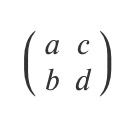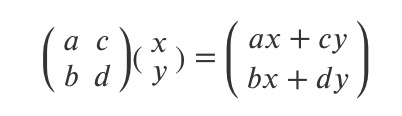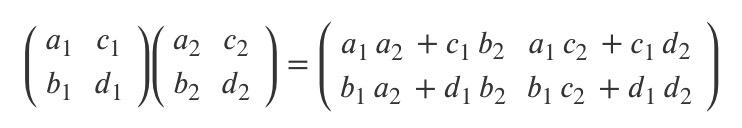Time Flying### transform-function

A linear transformation function is described as a `2 x 2` matrix, like this:The transformation function is applyied to an element by using matrix multiplication. Thus each cordinate changes is based on the matrix value.If several transformation work on the the same element, it can be writen in a row.### matrix()

The result of matrix is equal to `<transform-function>`.

`matrix(a, b, c, d, tx, ty)` is equal like this:

------- 占位 --------